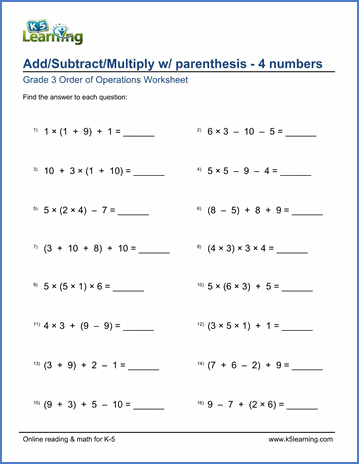# Order of Operations Worksheets

## Order of operations / PEMDAS worksheets

Our order of operations worksheets vary in difficulty by varying the number of terms, the included operations and whether parenthesis are included. The worksheets are categorized by grade.

Topics include:

## Grade 3 order of operations worksheets

• Add / subtract with parenthesis: 3-6 terms
• Add / subtract / multiply with no parenthesis
• Add / subtract / multiply with parenthesis: 4-6 terms

## Grade 4 order of operations worksheets

• Add / subtract with parenthesis: 5-6 terms
• Add / subtract / multiply with parenthesis: 5-6 terms
• Add / subtract / multiply / divide with parenthesis: 5-6 terms

## Grade 5 order of operations / PEMDAS worksheets

• 4 operations with parenthesis
• PEMDAS including exponents
• PEMDAS with exponents and nested parenthesis

## Order of operations: drills & practice

• Add & subtract multiple terms with parenthesis
• 4 operations with parenthesis
• PEMDAS (4 operations with exponents and parenthesis)

## Related topics